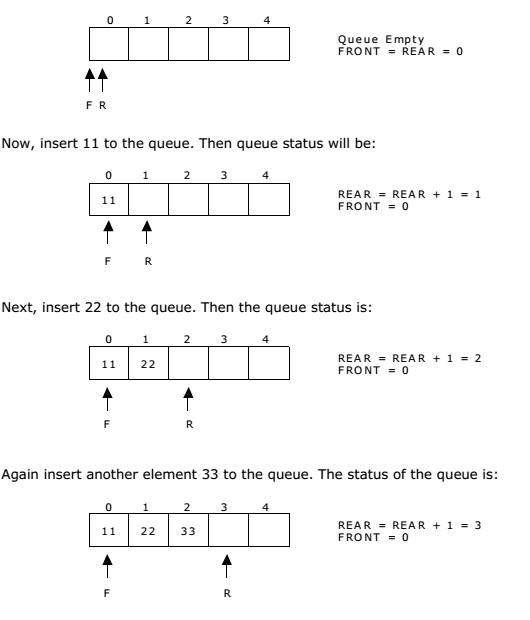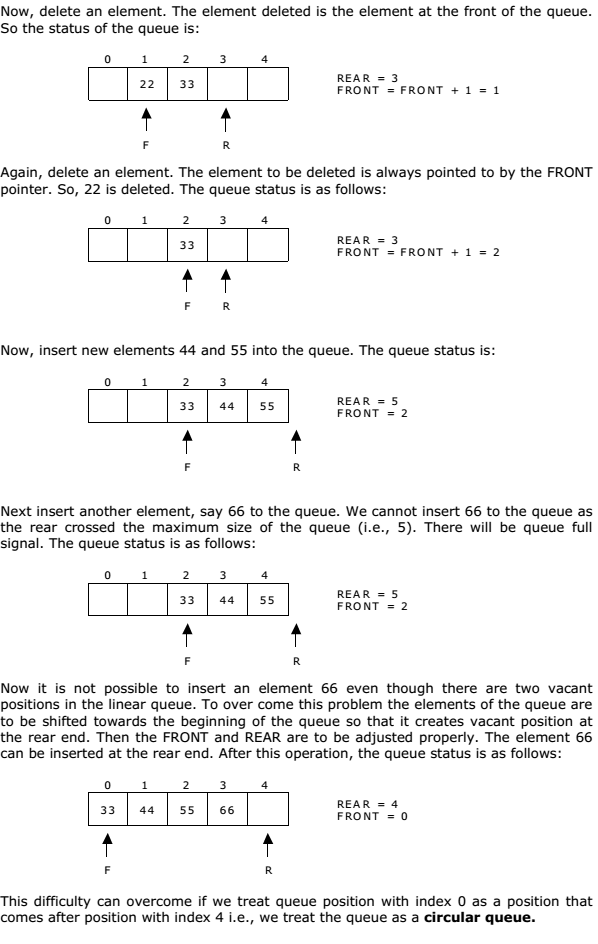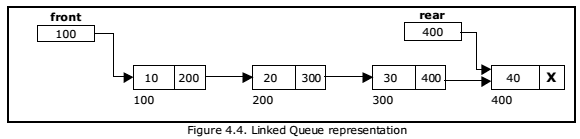A queue is another special kind of list, where items are inserted at one end called the rear and deleted at the other end called the front. Another name for a queue is a “FIFO” or “First-in-first-out” list.

The operations for a queue are analogues to those for a stack, the difference is that the insertions go at the end of the list, rather than the beginning. We shall use the following operations on queues:

• enqueue: which inserts an element at the end of the queue.
• dequeue: which deletes an element at the start of the queue.

Representation of Queue:

Let us consider a queue, which can hold maximum of five elements. Initially the queue is empty.Queue operations using array:

In order to create a queue we require a one dimensional array Q(1:n) and two variables front and rear. The conventions we shall adopt for these two variables are that front is always 1 less than the actual front of the queue and rear always points to the last element in the queue. Thus, front = rear if and only if there are no elements in the queue. The initial condition then is front = rear = 0. The various queue operations to perform creation, deletion and display the elements in a queue are as follows:

1. insertQ(): inserts an element at the end of queue Q.
2. deleteQ(): deletes the first element of Q.
3. displayQ(): displays the elements in the queue.

We can represent a queue as a linked list. In a queue data is deleted from the front end and inserted at the rear end. We can perform similar operations on the two ends of a list. We use two pointers front and rear for our linked queue implementation.

The linked queue looks as shown in figure 4.4:Applications of Queue:

1. It is used to schedule the jobs to be processed by the CPU.
2. When multiple users send print jobs to a printer, each printing job is kept in the printing queue. Then the printer prints those jobs according to first in first out (FIFO) basis.
3. Breadth first search uses a queue data structure to find an element from a graph.

You may be interested in:
Data Structures and Algorithms – MCQs.
Data Structures and Algorithms Online Tests